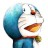# 杰拉斯的博客

## [ACM_ZJUT_2012]勘探油田杰拉斯 | 时间：2012-03-28, Wed | 5,872 views

### 勘探油田

Time Limit:1000MS Memory Limit:32768K

```1 1
*
3 5
*@*@*
**@**
*@*@*
1 8
@@****@*
5 5
****@
*@**@
*@**@
@@@*@
@@**@
0 0
```

```0
1
2
2
```

#### Source

ZJUT2012

```#include<iostream>
#include<queue>

using namespace std;

struct Pos{
int x;
int y;
Pos(int _x, int _y):x(_x),y(_y){};
};

int offset = {{-1, -1}, {-1, 1}, {1, -1}, {1, 1}, {-1, 0}, {1, 0}, {0, -1}, {0, 1}};

int main(){
int m, n, i, j;
while(cin >> m >> n){
if(m == 0 && n == 0)
break;
int num = 0;
char **a = new char*[m];
for(i = 0; i < m; ++i){
a[i] = new char[n];
cin >> a[i];
}
queue<Pos> q;
for(i = 0; i < m; ++i){
for(j = 0; j < n; ++j){
if(a[i][j] == '@'){
q.push(Pos(i, j));
while(!q.empty()){
Pos p = q.front();
a[p.x][p.y] = 'o';
q.pop();
for(int k = 0; k < 8; ++k){
int x = p.x + offset[k];
int y = p.y + offset[k];
if(x >= 0 && x < m && y >= 0 && y < n && a[x][y] == '@'){
q.push(Pos(x, y));
}
}
}
++num;
}
}
}
cout << num << endl;
}
return 0;
}
```